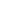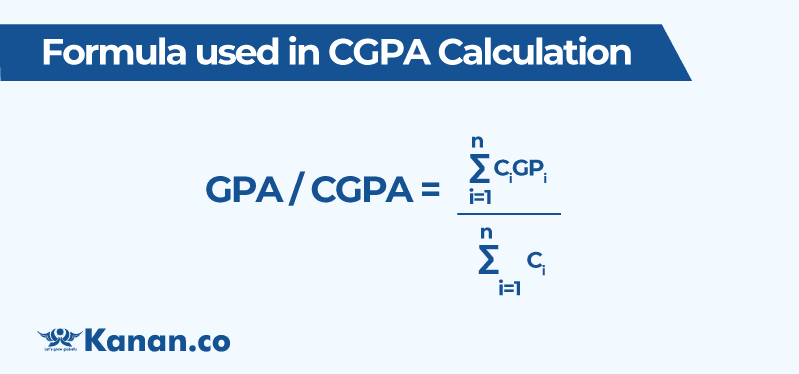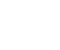Tejaswini Kota

updated on 24-02-2023# Anna University CGPA Calculator## GPA Calculator

Anna university regulation 2021

Subject 1

## CGPA Calculator

Anna university regulation 2021

Select Semesters

Students can get their accurate CGPA score through our Anna University CGPA calculator. Grade calculation is an important aspect that reflects your academic performance and is used as tools to get admission to your desired universities.

To calculate CGPA all you need is your GPA score which is analysed using your concerned subject grade and credit points. Know your gpa score details as well through our Anna university GPA calculator and Anna University CGPA calculator. This article will guide you in gaining clear information about how to calculate CGPA, anna university latest grading system details etc.

## What is CGPA and GPA?

Cumulative grade point average (CGPA) is the average grade point obtained by the students at the end of their academic program. The Anna University CGPA calculator gives results by adding the total grade points of all the subjects and then dividing it by the total number of subjects credit points.

 CGPA = (Sum of the ( grade points * credit point) for each subject )/ (sum of the credit points)

Grade Point Average (GPA) is the average of grade points obtained in one(single) semester. The Anna University GPA calculator calculates the ratio of sum of the product of course credits and grade points to the total number of course credits in a semester.

 GPA = ∑ (credit gained × grade point) / total credits earned

## Formula used in CGPA Calculation?

The formula used to evaluate and calculate CGPA is as follows:where

n is number of all courses successfully cleared during the particular semester in the case of GPA

GPi is the point corresponding to the grade obtained for each course

Ci is the number of Credits assigned to the course

## Details of grading system followed at Anna University for regulation 2021

Grades are awarded to the students based on the marks they scored that lie within a range of 0 to 100. Anna University has revised their grading system and information related to the updated grade system for regulation 2017 & 2021 is given below.

 Grade Letters Corresponding grade points Marks range O (Outstanding) 10 91-100 A+ (Excellent) 9 81-90 A (Very good) 8 71-80 B+ (Good) 7 61-70 B (Average) 6 51-60 C (Satisfactory) 5 <50 RA (Re-Appearance) 0 - SA (shortage of attendance) 0 - W (Withdrawal) 0 -

Note: To get distinction, students should get no less than 8.5 CGPA score without any arrears/backlogs in all the semesters. Students should score at least 6.5 CGPA to get First class

## How to calculate CGPA Manually?

Though the results are mentioned in your scorecard,many students do not know how to calculate CGPA. Here, through an example we have explained clearly so that students can understand how CGPA is calculated.

Example: The result details of semester-1 and semester-2 of a PG course is given below. calculate the total CGPA that student gained in that course.

For Semester-1

 List of subject Grade letter Credits for subject subject-1 O 3 subject-2 A+ 3 subject-3 O 3

For Semester-2

 List of subject Grade letter Credits for subject subject-A O 3 subject-B O 3 subject-C O 3

Step-1: Now let’s calculate average grade points and total credits for semester-1 & 2 separately

From the grading system table given above-
grade point for “O”=10 and For  “A+”= 9

For Semester-1

 List of subject Grade letter Grade point Credits for subject Subject-1 O 10 3 subject-2 A+ 9 3 subject-3 O 10 3

=(10+9+10)/3
=29/3
=9.66

Total credits = 3+3+3= 9

TOTAL GRADE POINT for semester-1 = AVERAGE GRADE POINT X TOTAL CREDITS
= 9.66 x 9
= 86.94

For Semester-2

 List of subject Grade letter Grade point Credits for subject subject-A O 10 3 subject-B O 10 3 subject-C O 10 3

=(10+10+10)/3
=30/3
=10

Total credits = 3+3+3= 9

TOTAL GRADE POINT for semester-2 = AVERAGE GRADE POINT X TOTAL CREDITS
= 10 x 9
= 90

Step-2: CGPA calculation

 Total CGPA = (SUM OF TOTAL GRADE POINTS) / (SUM OF TOTAL CREDITS)

CGPA = (86.94+90) / (9+9)
= 176.9 / 18
= 9.83
Hence, the final CGPA result = 9.83

## CGPA to Percentage Conversion

CGPA score can be converted to percentage by multiplying the CGPA value with 10.

 Percentage= CGPA x 10

From the above example CGPA score is 9.83
percentage= 9.83 x 10 = 98.3%

To know more about CGPA and its conversion to percentage  please read how to convert CGPA to percentage

##### Key Takeaways:
• Cumulative grade point average and grade point average both indicate the weightage of marks secured by the student during their course program.
• GPA is calculated for a single semester while CGPA is calculated for the complete course duration(all semesters combined).

## FAQs of Anna University CGPA calculator

### 1. How does Anna University calculates CGPA?

The overall CGPA for Anna university is calculated by adding total grade points of all the subjects and then dividing it by total number of subject credits. GPA is the ratio of sum of product of course credits and grade points to the sum of course credits earned in a single semester.

### 2. How to convert Anna University CGPA to percentage?

The Anna university CGPA can be converted into percentage by multiplying the CGPA score by 10. For example, if your CGPA score is 8.3 , then the percentage is
Percentage = 8.3 x 10 = 83%.

### 3. How Anna University CGPA Calculator works?

The anna university cgpa calculator gives results by adding total grade points of all the subjects and then dividing it by total number of credits assigned to the course.

### 4. How Anna University GPA calculator works?

The Anna university GPA calculator calculates GPA for each semester. It is computed as the ratio of sum of product of course/subject credits and grade points to the sum of course credits earned in a single semester.

#### Recent posts from Tejaswini KotaTejaswini KotaTejaswini Kota

IELTS Score for Germany : Top Universities Score RequirementTejaswini Kota

Canada vs Germany: Which Country is suitable for Indian students?Tejaswini Kota

Anna University CGPA CalculatorTejaswini Kota

SOP for PhDTejaswini Kota

Salary of Software engineer in Canada, 2023A truly global higher education partner to learners and education institutions Support learners at every point in their global education journey with reliable services, products and solutions.

Kanan Int EdTech Inc

229, Yonge Street Suite 406 Toronto Ontario, Canada M5B 1N9

Kanan International Pvt. Ltd.+91 63570 12000admissions@kanan.co